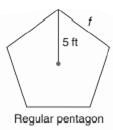Chapter 11.CR, Problem 4CR### Elementary Geometry for College St...

6th Edition
Daniel C. Alexander + 1 other
ISBN: 9781285195698

#### Solutions

Chapter
Section### Elementary Geometry for College St...

6th Edition
Daniel C. Alexander + 1 other
ISBN: 9781285195698
Textbook Problem
1 views

# In Exercises 1 to 4, state the ratio needed, and use it to find the measure of the indicated line segment to the nearest tenth of a unit.To determine

To find:

The measure of the indicated line segment to the nearest tenth of a unit.

Explanation

Procedure used:

In any right triangle ABC with mA=θ we have the following ratios.

sinθ=OppositeHypotenuse

Calculation:

Given:

The above figure is a pentagon with radius r=5 ft.

Let us name the given figure of pentagon as ABCDE.

Now,

OC=r=5 ft, and

CD= ft.

Calculate the angle subtended between the radii.

Now, the angle between the radii OC and OD is given by

=360°5

=72°

Thus,

mCOD=72°

### Still sussing out bartleby?

Check out a sample textbook solution.

See a sample solution

#### The Solution to Your Study Problems

Bartleby provides explanations to thousands of textbook problems written by our experts, many with advanced degrees!

Get Started

#### Find the median for the following set of scores: 1, 9, 3, 6, 4, 3, 11, 10

Essentials of Statistics for The Behavioral Sciences (MindTap Course List)

#### In Exercises 1-22, evaluate the given expression. 6!4!2!

Finite Mathematics for the Managerial, Life, and Social Sciences

#### In problems 23-58, perform the indicated operations and simplify. 41.

Mathematical Applications for the Management, Life, and Social Sciences

#### The angle θ at the right has cosine:

Study Guide for Stewart's Multivariable Calculus, 8th

#### True or False: is a convergent series.

Study Guide for Stewart's Single Variable Calculus: Early Transcendentals, 8th